# Table of three Derivatives and Definition#Printable Table of 3 Definition & Derivatives

An online printable table of derivatives and definition chart.

# Table of Derivatives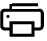Power of X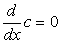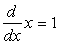Exponential / Logarithmic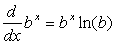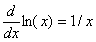Trigonometric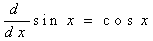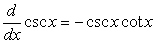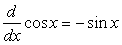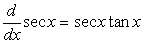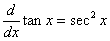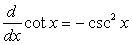Inverse Trigonometric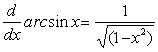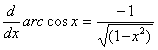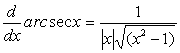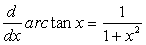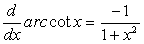Hyperbolic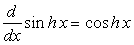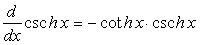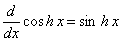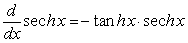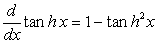# Definition1st Derivative
The derivative of a function describes how changes in one variable are related to changes in another. One representation of this concept in geometry is in the slope of the tangent to a curve. The derivative of a function y = f(x) is defined as: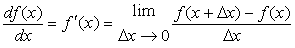2nd Derivative
If y = f(x) is a differentiable function, then differentiation produces a new function y' = f'(x) called the first derivative of y with respect to x. If y' = f'(x) is in turn a differentiable function, then its derivative, df'(x)/dx, is called the second derivative of y with respect to x.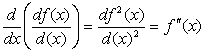3rd Derivative
Likewise, the third derivative is the derivative of the second derivative.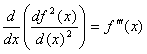General Form
In general, the nth derivative of f is denoted by f (n) and is obtained from f by differentiating n times, as such: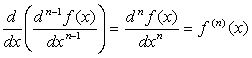##Age Calculators ►

Horse Age Calculator , Cow Age Calculator , Cat Age Calculator , Dog Age Calculator, Human Age Calculator 100 Days Old Calculator Date Difference Calculator